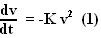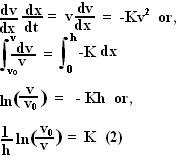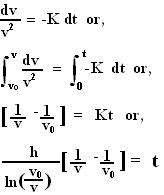## Saturday, November 24, 2007

### Irodov Problem 1.101

Since the resistance to the bullet is proportional to the squre of its velocity, it deceleration must be proportional to the square of its velocity. In other words,Here, K is a positive proportionality constant. Let x represent the distance travelled by the bullet in the plank. From (1) we have,To calculate time the bullet took to travel through the plank we take the following approach. From (1) we have,